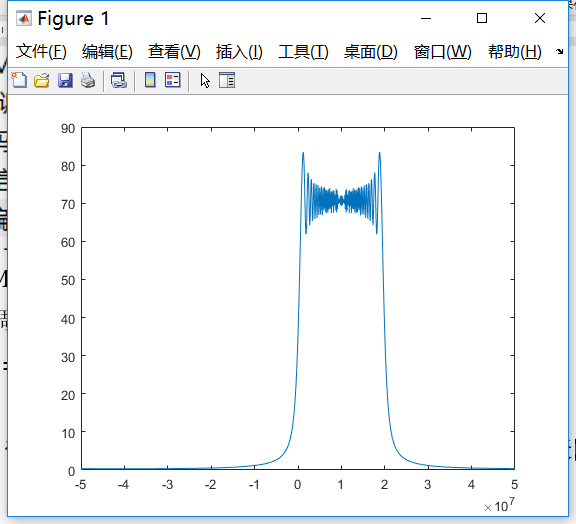• clear; B=17e6;%带宽 T=10e-6;%脉宽 f0=2e6;%中心频率 fs=100e6;%载频 N=3000;%采样点 t=linspace(0,0.5*T,N);...% 线性调频信号 plot(t,real(x)) ff=-fs/2:fs/N:fs/2-fs/N; plot(ff,abs(fftshift(fft(x)))) ...
clear;
B=20e6;%带宽
T=10e-6;%脉宽
f0=10e6;%中心频率
fs=100e6;%采样频率
N=round( T/(1/fs));%采样点数
t=linspace(-0.5*T,0.5*T,N);%时间
K=B/T;%调频斜率
x=exp(1j*2*pi*(f0*t+K*t.^2/2));%信号
% 线性调频信号
plot(t,real(x))
ff=-fs/2:fs/N:fs/2-fs/N;
plot(ff,abs(fftshift(fft(x))))


关键的问题在于采样点数应该是计算出来的，而不是自己随便定的展开全文• 线性调频信号，LFM(chirp)信号，解决了雷达作用距离和距离分辨率之间的矛盾，运用广泛。对其进行软件仿真及进一步压缩处理，取其时域频域仿真波形。T=4e-6B=500e6K=B/T;f0=0;Fs=2*B;Ts=1/Fs;N=T/Ts;t=linspace(-T/2,...
线性调频信号，LFM(chirp)信号，解决了雷达作用距离和距离分辨率之间的矛盾，运用广泛。对其进行软件仿真及进一步压缩处理，取其时域频域仿真波形。T=4e-6B=500e6K=B/T;f0=0;Fs=2*B;Ts=1/Fs;N=T/Ts;t=linspace(-T/2,T/2,N);St=exp(j*2*pi*f0*t+j*pi*K*t.^2);subplot(211);plot(t*1e6,real(St));xlabel('Time in u sec');title('Real part of chirp signal');grid on;axis tight;subplot(212);freq=linspace(-Fs/2,Fs/2,N);plot(freq*1e-6,fftshift(abs(fft(St))));xlabel('Frequency in MHz');title('Magnitude spectrum of chirp signal');grid on;axis tight;ht=exp(-j*pi*K*t.^2);Z=conv(St,ht);figure;plot(abs(Z))在时域上时间的范围0到T，频域对应的为0到fs。同时，在匹配滤波，及卷积的同时，出现了点的偏移，需要处理。
展开全文• 通过MATLAB仿真线性调频信号，得出线性调频信号的实部虚部和时间关系，得出调频信号的时频关系，得出调频信号的频谱，得出调频信号的相位与时间的关系。
• 雷达入门必做：线性调频信号脉冲压缩仿真，用了两种方法进行脉冲压缩，分别是匹配滤波和扩展处理
• %% LFM线性调频信号%% fs=48e6; %采样率：fs=1.2B此时效果最好 T=2e-6; %脉宽：脉冲持续时间 B=40e6; %带宽：所包含的所有的频率成分 k=B/T; %调频斜率 n=round(Tfs); %采样点个数，round是取整 t=linspace(-T/2,T/2...
clear all;
%%  LFM线性调频信号%%
fs=48e6;       %采样率：fs=1.2B此时效果最好
T=2e-6;         %脉宽：脉冲持续时间
B=40e6;         %带宽：所包含的所有的频率成分
k=B/T;          %调频斜率
n=round(Tfs);  %采样点个数，round是取整
t=linspace(-T/2,T/2,n);     %步长取点
y=exp(1jpikt.^2);        %信号
c = 3e8;
R0 = 50;    %定点
sr = exp(jpik*(t-2*R0/c).^2);		%定点回波信号
figure;
subplot(2,2,1);plot(t,real(y));        %real是实部，imag是虚部，两者偏转90°，abs是幅值
title(‘LFM信号时域’);
xlabel(‘t/s’);
ylabel(‘幅度’);
fft_y=fftshift(fft(y));     %傅里叶变换
f=linspace(-fs/2,fs/2,n);
subplot(2,2,2);plot(f,abs(fft_y));
title(‘LFM信号频谱’);
xlabel(‘f/HZ’);
ylabel(‘幅度’);
subplot(2,2,3);plot(t,real(sr));
fft_sr=fftshift(fft(sr));     %傅里叶变换
f=linspace(-fs/2,fs/2,n);
subplot(2,2,4);plot(f,abs(fft_sr));


展开全文• 本程序是线性调频信号matlab仿真。包括信号时域频域分析，去斜率处理等。
• Chirp信号的简介 Chirp信号是一个典型的非平稳信号，在通信、声纳、雷达等领域具有广泛的应用。 这是一个matlab对chirp信号的仿真实验
• 用于USRP发射的带占空比的LFM线性调频信号matlab源程序(保存为.txt文本信号用于labview读取)
• 根据不同的窗函数编制matlab程序产生不同的非线性调频信号 根据不同的窗函数编制matlab程序产生不同的非线性调频信号
• LFM线性调频信号脉冲多普勒雷达matlab仿真，MTD，MTI。
• 摘 要：本论文对目前在雷达信号处理系统中应用较为广泛的脉冲压缩技术进行了...其次对线性调频信号的匹配滤波器进行了研究，并分析比较了在白噪声下通过匹配滤波器的线性调频信号的波形；最后在MATLAB6.5平台上对线...
摘 要：本论文对目前在雷达信号处理系统中应用较为广泛的脉冲压缩技术进行了介绍，主要是线性调频的脉冲压缩信号。首先对脉冲压缩的概念以及雷达的工作原理进行了介绍，其次介绍了线性调频脉冲压缩技术的基本原理，以此为基础仿真生成了线性调频信号，并对其频域特性进行了详细的分析；其次对线性调频信号的匹配滤波器进行了研究，并分析比较了在白噪声下通过匹配滤波器的线性调频信号的波形；最后在MATLAB6.5平台上对线性调频脉冲压缩雷达进行了仿真。利用线性调频信号具有大带宽、长脉冲的特点，宽脉冲发射已提高发射的平均功率并保证足够的作用距离；而接受时采用相应的脉冲压缩算法获得窄脉冲已提高距离分辨率，较好的解决了雷达作用距离和距离分辨率之间的矛盾。57708毕业论文关键词：雷达仿真，线性调频，脉冲压缩，匹配滤波器，白噪声Abstract：This paper mainly introduced the linear frequency modulated pulse compression signal, which has been widely applied in radar signal processing system and pulse compression technology. Firstly, the concept of pulse compression and the working principle of radar are introduced in this paper, and it introduces the basic principle of the linear frequency modulation pulse compression technology, which the simulation to generate the linear frequency modulation signal is based on, and analyzes the frequency domain characteristics in detail; Secondly, it mainly do research on the matched filter of linear frequency modulation signal, and compares with matched filter of linear frequency modulation signal waveform in the white noise; Finally, it simulates  the linear frequency modulation pulse compression radar in MATLAB6.5 platform. The characteristics of long pulse width of pulse firing has raised the average power to ensure enough emission range; and the corresponding pulse compression algorithm to when accepting obtain the narrow pulse has improve the range resolution, they are all better solved the contradiction between the radar range and range resolution.Key words：Radar simulation，chirp，pulse compression，Matched filter目录1 引言41.1 脉冲压缩的概念41.2 雷达工作原理52  设计原理72.1 匹配滤波器原理72.2 线性调频脉冲信号82.3 线性调频脉冲压缩的基本原理113  雷达LFM脉冲压缩设计143.1 线性调制信号和噪声的生成143.2 设计结果163.3 设计分析19结论21参考文献22致谢23附录241 引言随着雷达技术的迅猛发展，对雷达的作用距离、分辨能力和测量精度等的要求也越来越高。我们知道按雷达信号的分辨理论。在保证一定信噪比并实现最佳处理的前提下，测量精度和分辨力对信号形式的要求完全一致：测距精度和距离分辨力主要取决于信号的频率结构，他要求信号具有大的带宽、测速精度和速度分辨力取决于信号的时间结构，他要求信号具有大的时宽。因此，理想的雷达信号应具有大的时宽带宽乘积。大时宽保证了速度分辨力，大带宽则保证了距离分辨力。但是大时宽和大带宽往往不可兼得。也就是说，测距精度和距离分辨力以及测速精度和速度分辨力之间存在着不可调和的矛盾。 线性调频脉冲信号压缩的原理及MATLAB仿真程序:http://www.lwfree.com/zidonghua/lunwen_62530.html
展开全文• 线性调频信号的数学表达式： 其中，t是时间变量，单位为秒（s）；T为脉冲持续时间（周期）；K是线性调频率，单位是Hz/s； 角度（单位为弧度）表达式： ...
• 这个代码是一个matlab编写的代码，他说明了LFM的模糊函数是怎么一回事
• 【实例简介】里面有关于实现matlab的算法以及分析处理山国科技记文在线分布的时频平面作直线积分投影的变换,统称对信号作变换在分布的时频平面里惯用轴的截距和斜率为参数表小直线。因此,当需要沿作直线积分时,可将...
• matlab仿真，线性调频信号，经过匹配滤波器仿真结果
• 基于MATLAB线性调频信号的仿真,很好的学习资料，大家可以参考下
• 线性调频信号的数学分析 代码： %%-----------------------------------------------------------------------------------------------------------%% %%说明：调用此函数可以输出线性调频信号余弦表达式下的...fft
• 基于Matlab的DDS线性调频信号的仿真应用、电子技术,开发板制作交流DDS 调频信号 FPGA
• matlab 线性调频信号匹配滤波器 点目标回波 距离分辨 提高性噪比匹配滤波
• 基于matlab线性调频信号的仿真 I存档编号________ 基于 MATLAB 的线性 调频信号的仿真教学学院 届 别 专 业 学 号 指导教师 完成日期 I 内容摘要：线性调频信号是一种大时宽带宽积信号。线性调频信号的 相位谱具有...
• 超详细的线性调频信号的模糊函数matlab程序，博主亲自撰写，确认无误程序
• 对于回波信号进行脉冲压缩和频域加窗处理，用于雷达信号仿真
• matlab仿真线性调频信号代码 包括绘制时域波形和频域波形
• 文章目录线性调频与脉冲压缩1 线性调频信号1.1 线性调频信号（时域）1.1.1 线性调频信号模型1.1.2 线性调频信号时域仿真1.2 线性调频信号（频域）1.2.1 驻定相位原理（POSP）1.2.2 线性调频信号频谱1.2.3 线性调频...算法 自动驾驶
• 1.利用驻定相位原理推到线性调频信号的频谱。 2.在Matlab中对直接fft和驻定相位原理推导的结果进行比较仿真。...

# 线性调频信号matlabmatlab 订阅